cancel
Showing results for
Did you mean:Helper III

## How to calculate average based on levels (Country -> City)

Hi,

I’m facing the issue is below, while average is working in city level but not working as country level.

My data is look like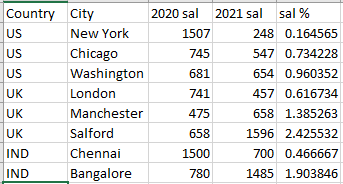My report design,

level – Country -> City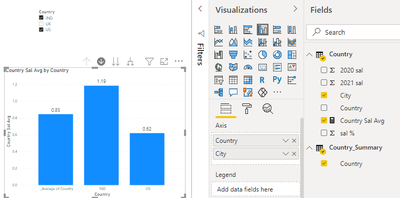Here Average is not working as expected in country level for example,

IND – 1.19 and US 0.62, actually, Overall average I’m trying to calculated sum of sales/no of country.

I would expect Overall average is (1.19 + 0.62) / 2 = 0.90 but it’s returned 0.85.

I don’t know, what is the issue. Someone suggests me.

Fyi,  it is working fine in city level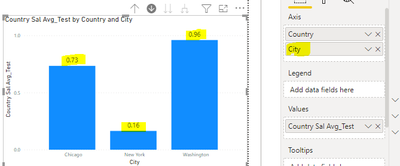regards,

Ram

2 ACCEPTED SOLUTIONSCommunity Support

According to your description and sample picture, I don’t think the Overall average is simply calculated as you expected as (1.19 + 0.62) / 2 = 0.90 because “US” has three cities and “Ind” has two cities, so the US occupies a greater weight in the calculation of the average, it’s like (0.16+0.73+0.96+0.46+1.90)/5=0.85.Therefore, I think the average calculation you used is correct and there’s no need for you to make any change.

If you still want to get the calculation like “0.90”, you can try this measure:

``````Average =

var _US=

IF("US" in SELECTCOLUMNS('Table',"1",[Country]),AVERAGEX(FILTER(ALL('Table'),[Country]="US"),[sal%]),0)

var _UK=

IF("UK" in SELECTCOLUMNS('Table',"1",[Country]),AVERAGEX(FILTER(ALL('Table'),[Country]="UK"),[sal%]),0)

var _IND=

IF("IND" in SELECTCOLUMNS('Table',"1",[Country]),AVERAGEX(FILTER(ALL('Table'),[Country]="IND"),[sal%]),0)

var _sum=

_US+_UK+_IND

return

DIVIDE(_sum,DISTINCTCOUNT('Table'[Country]))``````

And you can get what you want, like this: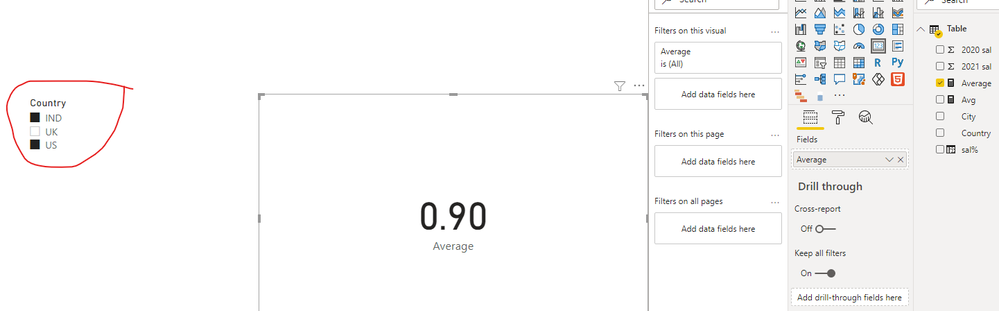Best Regards,

Community Support Team _Robert Qin

If this post helps, then please consider Accept it as the solution to help the other members find it more quickly.Community Support

According to your sample pbix file, I opened it and added the measure I posted above then changed the measure [Country Sal Avg] like this:

``````Country Sal Avg1 =
VAR __SelectedLabel = SELECTEDVALUE('Country_Summary'[Country])// 'Table 2'[Month])

RETURN
SWITCH(__SelectedLabel,
"_Average of Country", [Average],
CALCULATE(AVERAGE('Country'[sal %]), FILTER('Country', 'Country'[Country] = __SelectedLabel))
)``````

Then the average value in the column chart can display the value you wanted, like this: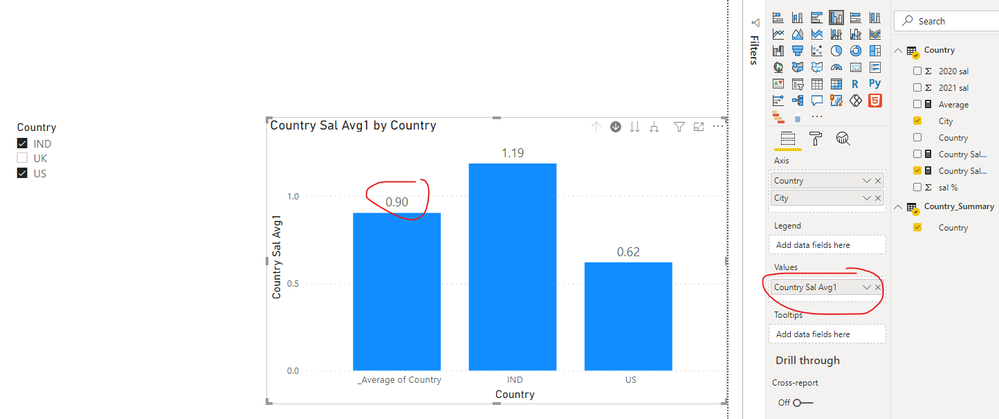And you can get what you want.

Best Regards,

Community Support Team _Robert Qin

If this post helps, then please consider Accept it as the solution to help the other members find it more quickly.

8 REPLIES 8Community SupportCommunity Support

According to your description and sample picture, I don’t think the Overall average is simply calculated as you expected as (1.19 + 0.62) / 2 = 0.90 because “US” has three cities and “Ind” has two cities, so the US occupies a greater weight in the calculation of the average, it’s like (0.16+0.73+0.96+0.46+1.90)/5=0.85.Therefore, I think the average calculation you used is correct and there’s no need for you to make any change.

If you still want to get the calculation like “0.90”, you can try this measure:

``````Average =

var _US=

IF("US" in SELECTCOLUMNS('Table',"1",[Country]),AVERAGEX(FILTER(ALL('Table'),[Country]="US"),[sal%]),0)

var _UK=

IF("UK" in SELECTCOLUMNS('Table',"1",[Country]),AVERAGEX(FILTER(ALL('Table'),[Country]="UK"),[sal%]),0)

var _IND=

IF("IND" in SELECTCOLUMNS('Table',"1",[Country]),AVERAGEX(FILTER(ALL('Table'),[Country]="IND"),[sal%]),0)

var _sum=

_US+_UK+_IND

return

DIVIDE(_sum,DISTINCTCOUNT('Table'[Country]))``````

And you can get what you want, like this:Best Regards,

Community Support Team _Robert Qin

If this post helps, then please consider Accept it as the solution to help the other members find it more quickly.Helper III

Thank you for your quick response..

I have downloaded and verified the .pbix file, the average is showing is correct. but, the overall country average column is not shown in here,

I would the output is link, Average of Country level -> Average of City levelif you can possible, please add the column or give suggestion for this.

thanks,

RamCommunity Support

According to your picture, I’m wondering how can you add an “Average of country” column in the left of your column chart. The measure I created can only get one average value based on the country slicer. Would you like to post your pbix file(without sensitive data)?

Thanks very much!

Best Regards,

Community Support Team _Robert Qin

If this post helps, then please consider Accept it as the solution to help the other members find it more quickly.Helper III

I couldn't add .pbix file here. so, I attached file in google drive and link is below,

please check and let me know if any issueCommunity Support

According to your sample pbix file, I opened it and added the measure I posted above then changed the measure [Country Sal Avg] like this:

``````Country Sal Avg1 =
VAR __SelectedLabel = SELECTEDVALUE('Country_Summary'[Country])// 'Table 2'[Month])

RETURN
SWITCH(__SelectedLabel,
"_Average of Country", [Average],
CALCULATE(AVERAGE('Country'[sal %]), FILTER('Country', 'Country'[Country] = __SelectedLabel))
)``````

Then the average value in the column chart can display the value you wanted, like this:And you can get what you want.

Best Regards,

Community Support Team _Robert Qin

If this post helps, then please consider Accept it as the solution to help the other members find it more quickly.Helper III

Sorry for the multiple request,

I can get "Average Country" based on Country slicer and its working fine. Now, I want to add one more slicer for "City" then what I do? Can you give suggestion?

The report like,thanks,

RamCommunity Support

Would you like to post your sample data in the “Form” type so that we can just copy and paste it into our pbix file to help you in advance?

Best Regards,

Community Support Team _Robert Qin

If this post helps, then please consider Accept it as the solution to help the other members find it more quickly.